Courses

# Chapter Notes - Symmetry Class 7 Notes | EduRev

## Class 7 : Chapter Notes - Symmetry Class 7 Notes | EduRev

The document Chapter Notes - Symmetry Class 7 Notes | EduRev is a part of the Class 7 Course Mathematics (Maths) Class 7.
All you need of Class 7 at this link: Class 7

14. Symmetry

Line Symmetry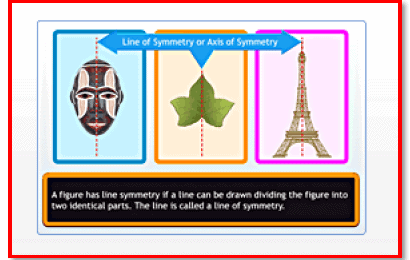A figure has line symmetry if a line can be drawn dividing the figure into two identical parts.

The word symmetry comes from the Greek word symmetros, which means even.

A figure has line symmetry if a line can be drawn dividing it into two identical parts. The line is called the line of symmetry or axis of symmetry.

Line symmetry is also known as reflection symmetry because a mirror line resembles the line of symmetry, where one half is the mirror image of the other half. Remember, while looking at a mirror, an object placed on the right appears to be on the left, and vice versa.

For a line segment, the perpendicular bisector is the line of symmetry.

For an equilateral triangle, the bisectors of the internal angles are the lines of symmetry.

For a square, the lines of symmetry are the diagonals and the lines joining the mid-points of the opposite sides.

The lines of symmetry of a rectangle are the lines joining the mid-points of the opposite sides.

The line of symmetry of an isosceles triangle is the perpendicular bisector of the non-equal side.

A scalene triangle, in which all the sides are of different lengths, doesn't have any line of symmetry.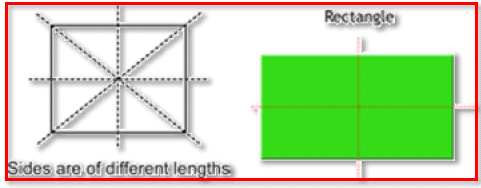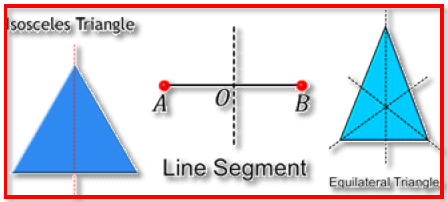Page 55

Regular polygon:

A polygon is said to be a regular polygon if all its sides are equal in length and all its angles are equal in measure. If a polygon is not a regular polygon, then it is said to be an irregular polygon.

Regular and irregular polygons have lines of symmetry. An equilateral triangle is regular because each of its sides has the same length, and each of its angles measures sixty degrees.

The number of lines of symmetry in a regular polygon is equal to the number of sides that it has.

A pentagon has five lines of symmetry.

Similarly, a regular octagon has eight sides, and therefore, it will have eight lines of symmetry, while a regular decagon has ten sides, so it will have ten lines of symmetry.

Irregular polygon:

Most irregular polygons do not have line symmetry. However, some of them do. Look at the rectangle and the isosceles triangle. A rectangle has two lines of symmetry, and an isosceles triangle has one line of symmetry.

Some letters have line symmetry.

Page 56

The letters A, B, C, D, E, I, K, M, T, U, V, W and Y have one line of symmetry.

The letter H overlaps perfectly both vertically and horizontally. So it has two lines of symmetry.

Similarly, the letter X has two lines of symmetry.

The letters F, G, J, L, N, P, Q, R, S and Z have no line of symmetry.

Rotational Symmetry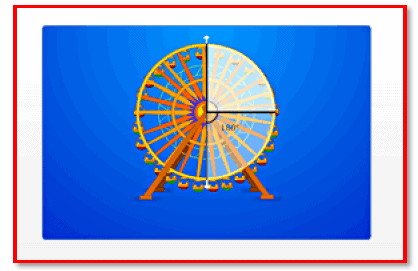Any object or shape is said to have rotational symmetry if it looks exactly the same...

Any object or shape is said to have rotational symmetry if it looks exactly the same at least once during a complete rotation through three hundred and sixty degrees.

During the rotation, the object rotates around a fixed point. Its shape and size do not change.

This fixed point is called the centre of rotation.

Rotation may be clockwise or anti-clockwise.

A full turn refers to a rotation of three hundred and sixty degrees.

A half turn refers to a rotation of one hundred and eighty degrees.

A quarter turn refers to a rotation of ninety degrees.

The angle at which a shape or an object looks exactly the same during rotation is called the angle of rotation.

Page 57

The order of rotational symmetry can be defined as the number of times that a shape appears exactly the same during a full 360orotation.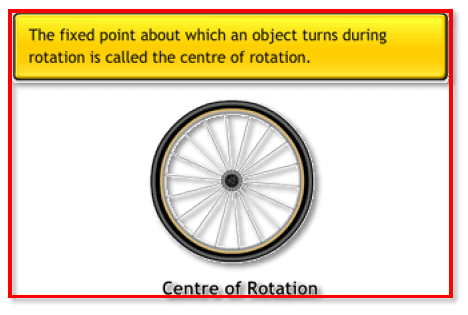The centre of rotation of a square is its centre. The angle of rotation of a square is 90 degrees, and its order of rotational symmetry is 4.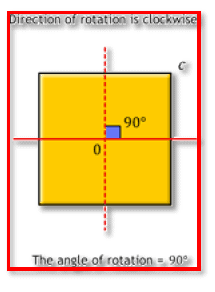The centre of rotation of a circle is the centre of the circle.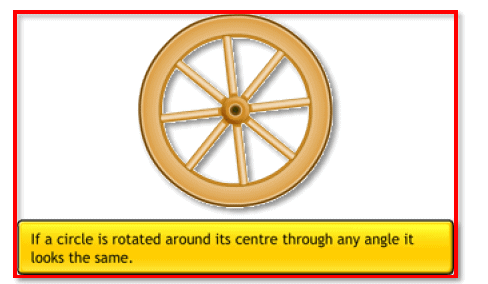There are many shapes that have only line symmetry and no rotational symmetry at all.

Some objects and shapes have both, line symmetry as well as rotational symmetry.

The Ashok Chakra in the Indian national flag has both, line symmetry and rotational symmetry.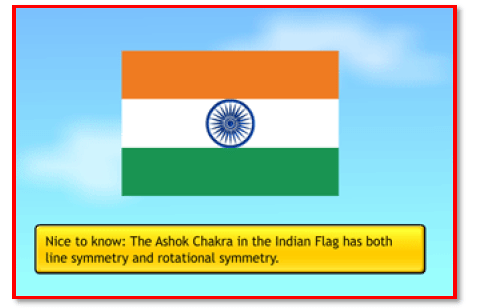Symmetry can be seen in the English alphabet as well.

The letter H has both line symmetry and rotational symmetry.

 Letter Line Symmetry Rotational Symmetry Z No Yes H Yes Yes 0 Yes Yes E Yes No N No Yes C Yes No A Yes No B Yes No
Offer running on EduRev: Apply code STAYHOME200 to get INR 200 off on our premium plan EduRev Infinity!

## Mathematics (Maths) Class 7

161 videos|237 docs|45 tests

,

,

,

,

,

,

,

,

,

,

,

,

,

,

,

,

,

,

,

,

,

;